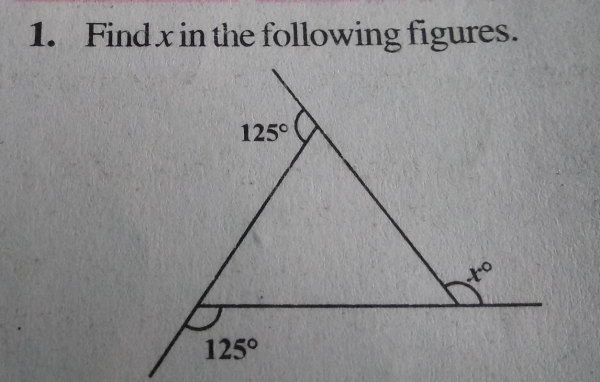# What is x in this figure?"

Given: The 2 exterior angles of a triangle are 125o.

To find: We have to find the value of the other exterior angle x.

Solution:

Now,

∠1 + 125o = 180o       (Linear pair of angles)

∠1 = 180o - 125o = 55o     .....(i)

∠2 + 125o = 180o       (Linear pair of angles)

∠2 = 180o - 125o = 55o     .....(ii)

∠3 + xo = 180o       (Linear pair of angles)

∠3 = 180o - xo     .....(iii)

and,

∠1 + ∠2 + ∠3 = 180o       (Sum of interior angles of a triangle)

Sustitute values from eq. (i), (ii) and (iii):

55o + 55o + 180o - xo = 180o

xo = 55o + 55o + 180o - 180o

xo = 110o